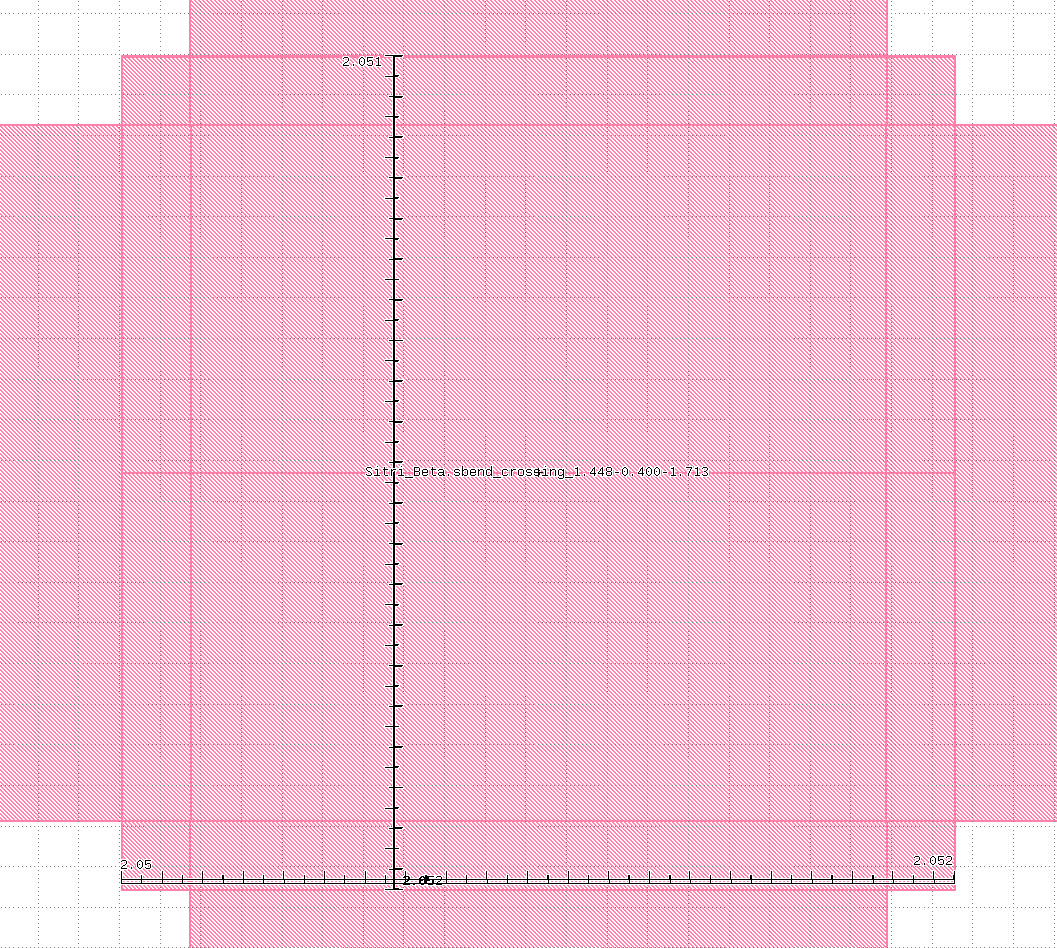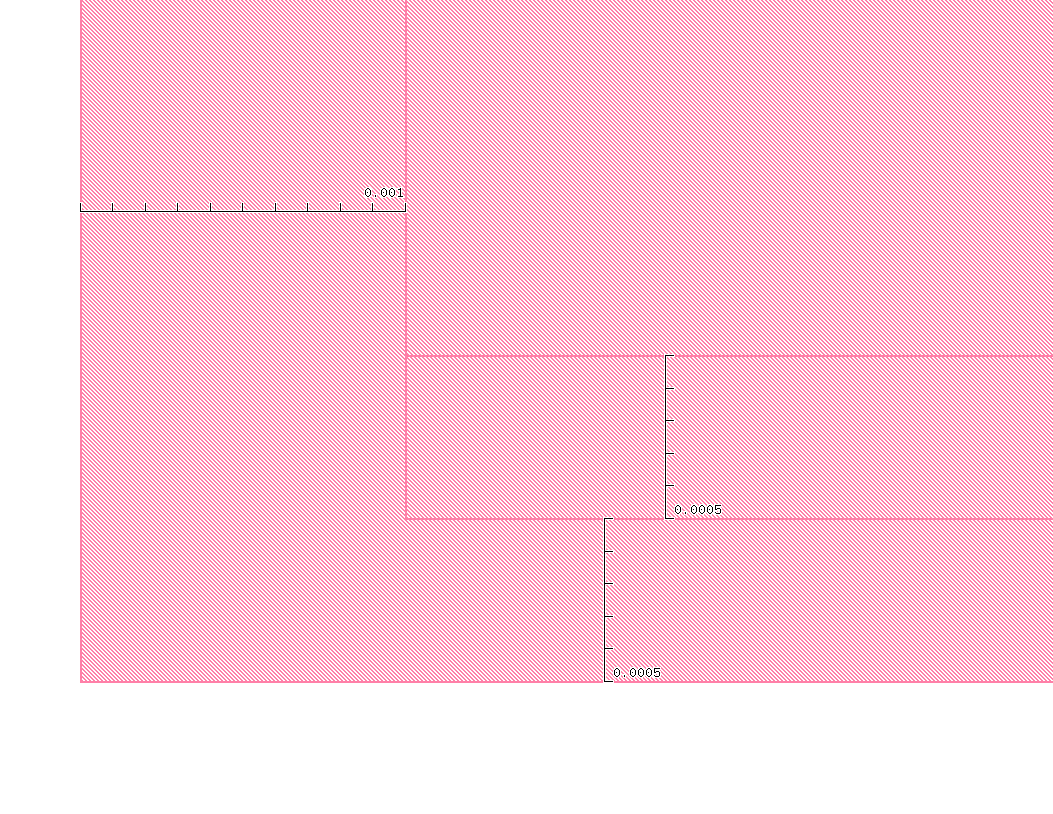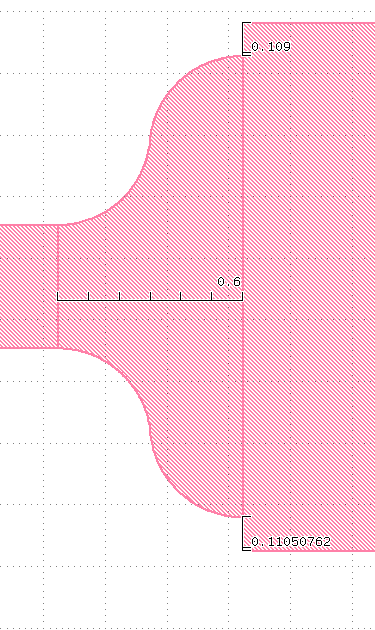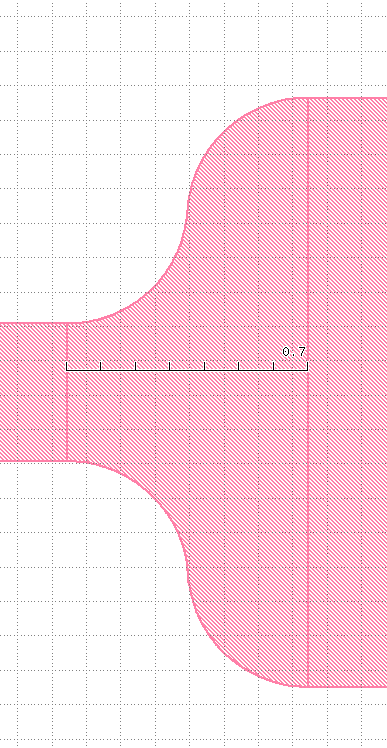# Why shapes formed by box and path has an error of 0.001um to 0.002um

edited August 2021

Hi，
I am using python scripting,
Using the following three method, I get three different shapes, although they should have the same structure.

``````    self.param("taper_l", self.TypeDouble, "The length of S sbend curve", default=1.448)
self.param("arm_length", self.TypeDouble, "The arm length", default=3.5)
........

l1 = self.taper_l / dbu
l2 = self.wg_length / dbu
l3 = self.arm_length / dbu
........
#method1
box4= Box(-0.5*(l3-l1), -0.5*(l3-l1), +0.5*(l3-l1),+0.5*(l3-l1))
shapes(LayerSi).insert(box4)
#method2
pin1 = Path([Point(-0.5*(l3-l1), 0),
Point(0.5*(l3-l1), 0)], (l3-l1))
shapes(LayerSi).insert(pin1)

#method3
pts7 = []
pts7.append(Point.from_dpoint(DPoint(-0.5*(l3-l1), -0.5*(l3-l1))))
pts7.append(Point.from_dpoint(DPoint(-0.5*(l3-l1), +0.5*(l3-l1))))
pts7.append(Point.from_dpoint(DPoint(+0.5*(l3-l1), +0.5*(l3-l1))))
pts7.append(Point.from_dpoint(DPoint(+0.5*(l3-l1), -0.5*(l3-l1))))

self.cell.shapes(LayerSi).insert(Polygon(pts7))
``````Only by the third method (polygon), I get the right structure.
Why shapes by box and path has a deviation？

I am very confused by this.
Could anyone give me some assitance?

• edited August 2021

Hi Weiling,

Why don't you use only DPoint, DBox and DPath??? No need to convert taper_l and arm_length to number of DBU equivalents...

`````` box = DBox.new(-0.5*(arm_length-taper_l), -0.5*(arm_length-taper_l), +0.5*(arm_length-taper_l),+0.5*(arm_length-taper_l))   #Ruby
``````

Now, let's say:

l1 = 1.447
l2 = 3.5

dbu = 0.001

in microns (DPoint, ...):

value = 0.5*(l2-l1) = 1.0265

This value is rounded to the nearest DBU point > (value/dbu).round*dbu = 1.027

in DBU units (Point, ...):

l1_dbu = l1/dbu = 1447.0

l2_dbu = l2/dbu = 3500.0

value_dbu = 0.5*(l2_dbu-l1_dbu) = 1026.5

This value is converted to an integer I guess as Point, Box, ... expect integer values > value_dbu.to_i = 1026

Notice the difference between .to_i and .round:

value_dbu.to_i = 1026

value_dbu.round = 1027

When using Path make sure the width is a multiple of 2*dbu, else the path edges will be off-DBU-grid as well...

Cheers,

Tomas

• @tomas2004
Hi, Tomas,
Thank you very much for your detailed response.
The method you provide does work. I know little about scripting in klayout. I just followed the way of others in siepic pdk.
(1)
Although the method you provide works well, is it wirted by ruby(I see you marked Ruby)?
Will this make the program unstable when the other parts seems to be written in python?

class sbend_crossing(pya.PCellDeclarationHelper):
def init(self):

``````    # Important: initialize the super class
super(sbend_crossing, self).__init__()
``````

(2)
I also find another strange thing.
I tried to add a structure made up by four arc bend.
Before, everything works well. When I continue to reduce the length of this structure to 0.6 um, I found that its height is not as expected, it is much smaller, about 0.1um, which could not be caused by dbu.
Can you see where the problem is?Normally, it should look like this:``````    self.param("wg_length", self.TypeDouble, "waveguide length", default=10)
self.param("taper_l", self.TypeDouble, "The length of S sbend curve", default=1.448)
self.param("wg_width", self.TypeDouble, "The width of port",default=0.4)
self.param("taper_w", self.TypeDouble, "The width of centre", default=1.714)
self.param("arm_length", self.TypeDouble, "The arm length", default=3.5)
.........
l1 = self.taper_l / dbu
l2 = self.wg_length / dbu
l3 = self.arm_length / dbu

w1 = self.wg_width / dbu
w2 = self.taper_w / dbu
.........
radius=((0.5 * l1) ** 2 + (0.5 * sbend_w) ** 2) / (2 * (0.5 *sbend_w))
angle=180 * asin((2 * (0.5 *l1) * (0.5 * sbend_w)) / (
(0.5 * l1) ** 2 + (0.5 * sbend_w) ** 2)) / pi
from math import pi, cos, sin

pts=[]
pts1 = []
pts2 = []
pts3= []
pts4 = []
from SiEPIC.utils import points_per_circle
circle_fraction = abs(angle) / 360.0
# npoints = int(points_per_circle(radius / 1000) * circle_fraction)
# # npoints = int(200 * circle_fraction)
# # if npoints == 0 or npoints ==1 :
# if npoints < 50 :
# npoints = 1
npoints = int(self.n * circle_fraction)
da = 2 * pi / npoints * circle_fraction  # increment, in radians
i_sum=[]
for i in range(0,  npoints + 1):
i_sum = i_sum + [i]

pts1.append(pya.Point.from_dpoint(pya.DPoint(
(radius * sin(i * da)), (radius * (1 - cos(i * da)))+w1/2)))
pts3.append(pya.Point.from_dpoint(pya.DPoint(2 * radius * sin(pi * angle / 180) +
(radius * sin(pi +i * da)),
-(w1 / 2 - 2 * radius * cos(pi * angle / 180) + (
radius * (1 - cos(pi + i * da)))) )))

isum1 = i_sum[::-1]
for i in range(len(isum1)):
pts2.append(pya.Point.from_dpoint(pya.DPoint(2 * radius * sin(pi * angle / 180) +
(radius * sin(pi + isum1[i] * da)),
w1/2-2 * radius * cos(pi * angle / 180) + (
radius * (1 - cos(pi + isum1[i] * da))))))
pts4.append(pya.Point.from_dpoint(pya.DPoint(
(radius * sin(isum1[i] * da)), -(radius * (1 - cos(isum1[i]* da))+ w1 / 2) )))

pts = pts1 + pts2 + pts3 + pts4

t9 = Trans(Trans.R0, -l3 - w2 / 2, 0)
self.cell.shapes(LayerSi).insert(Polygon(pts).transformed(t9))
``````
• Hi Weiling,

You cannot mix Ruby and Python within a script, but it looks quite similar, just drop the ".new":

``````box = DBox.new(-0.5*(arm_length-taper_l), -0.5*(arm_length-taper_l), +0.5*(arm_length-taper_l),+0.5*(arm_length-taper_l))
``````

Cheers,

Tomas

• @tomas2004 ,
Hi, Tomas,
Thank you very much for your respond.
I will check it.

Best regards!
Weiling